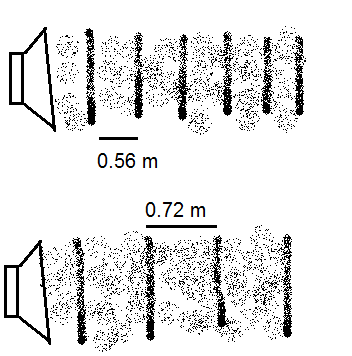Below is a diagram of the sound waves produced by two speakers, where the collection of dots ...

Question:

Below is a diagram of the sound waves produced by two speakers, where the collection of dots represent vibrating air molecules and the distance between each compression (i.e., the region where the molecules are closer together and a higher pressure) is also given. If you are standing near the speakers as they're playing, what beat frequency will you hear, assuming the speed of sound in air is {eq}343\ \dfrac ms {/eq}?Beats Frequency

When two sound waves of slightly different frequencies interfere, then we hear a periodic loud and soft sound called beats. The beat frequency (number of beats produced due to the interference of sound waves in one second) is equal to the difference in frequency of the two sounds that interfere in time to produce the beats

Given :

• The speed of sound is, {eq}v = 343 \ m/s {/eq}

(The wavelength of a sound wave is the distance between two consecutive compressions or two consecutive rarefactions)

• The wavelength of the sound produced from the first speaker is, {eq}\lambda_1 = 0.56 \ m {/eq}
• The wavelength of the sound produced from the second speaker is, {eq}\lambda_2 = 0.72 \ m {/eq}

The frequency of the sound produced by the first speaker will be,

{eq}\begin{align*} f_1 &= \dfrac{v}{\lambda_1} \\ f_1 &= \dfrac{343}{0.56} \\ f_1 &= 612.5 \ Hz \end{align*} {/eq}

The frequency of the sound produced by the second speaker will be,

{eq}\begin{align*} f_2 &= \dfrac{v}{\lambda_2} \\ f_2 &= \dfrac{343}{0.72} \\ f_2 &= 476.39 \ Hz \end{align*} {/eq}

The beat frequency which we will hear will be,

{eq}\begin{align*} f_b &= f_1 -f_2 \\ f_b &= 612.5 - 476.39 \\ f_b &= \boxed{ 136.1 \ Hz} \end{align*} {/eq}